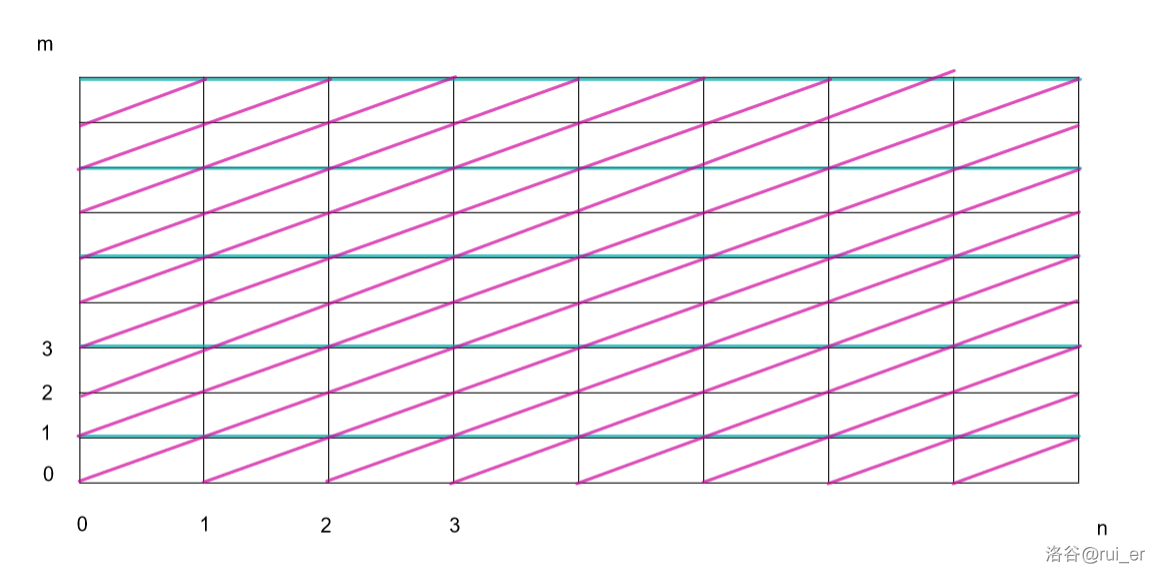rui_er 的博客rui_er 的博客

QAQ

题解 UVA1118 【Binary Stirling Numbers】

posted on 2020-09-26 10:36:08 | under 题解 |

\begin{aligned} S(n+1,m)=&S(n,m-1)+m\times S(n,m)&\\ S(n,m)=&S(n-1,m-1)+m\times S(n-1,m)&\\ S(n,m)\equiv&\begin{cases}S(n-1,m-1),&m\mod 2=0\\S(n-1,m-1)+S(n-1,m),&\operatorname{otherwise}\end{cases}&\pmod{2} \end{aligned}$$S(n,m)\mod 2=\begin{cases}1,&\left(n=m=0\right)\lor\left(\left(\frac{m+1}{2}-1\right)\operatorname{and}\left(n-m+\frac{m+1}{2}-1\right)=\frac{m+1}{2}-1\right)\\0,&\operatorname{otherwise}\end{cases}$$

//By: Luogu@rui_er(122461)
#include <bits/stdc++.h>
using namespace std;

#define f(a,b) (\
(a & b) == a\
)

int T, n, m;

int main() {
scanf("%d", &T);
while(T--) {
scanf("%d%d", &n, &m);
if(!n && !m) {
puts("1");
continue;
}
if(!n || !m || n < m) {
puts("0");
continue;
}
int a = (m + 1) / 2 - 1;
printf("%d\n", f(a, n-m+a));
}
return 0;
}